# Test: Electrical & Electronic Measurements- 2

## 20 Questions MCQ Test Mock Test Series for SSC JE Electrical Engineering | Test: Electrical & Electronic Measurements- 2

Description
Attempt Test: Electrical & Electronic Measurements- 2 | 20 questions in 12 minutes | Mock test for SSC preparation | Free important questions MCQ to study Mock Test Series for SSC JE Electrical Engineering for SSC Exam | Download free PDF with solutions
QUESTION: 1

### In which of the following do the measurements find their application?  i. Automatic control of processes &operations ii. Engineering experimental analysis iii. Monitoring of processes and operations

Solution:

In automatic control of processes and operations, measurement is required to control and provide the desired response

In engineering experimental analysis, measurement is required to find out the response of system to inputs

To monitor a system or process we need measurement

QUESTION: 2

### Induction Type Instruments can be employed for ________.

Solution:

Induction type of instruments ae used to measure AC quantities only. We can use these instruments as an ammeter, voltmeter, wattmeter and an energy meter.

QUESTION: 3

### The ratio of output change for a given measuring system is referred to as

Solution:

Sensitivity: It is the change in output to the unit change in input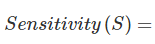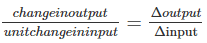Linearity:The property of an instrument, if output varies linearly according to input is known as linearity. It is the ability to produce the input characteristics symmetrically and linearly. The graphical relationship between input and output is a straight line. It is also a supporting characteristic of accuracy.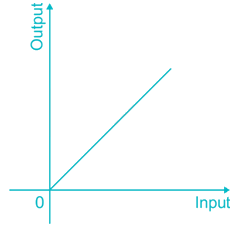QUESTION: 4

That would be the sensitivity of an ammeter, which requires a change of 3A in its coil to produce a change in deflection of the pointer by 6 mm?

Solution:

Sensitivity: It is the change in output to the unit change in input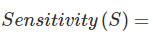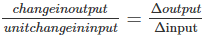Change in the output = 6 mm

Change in input = 3 A

Sensitivity (S) = 6/3 = 2 mm/A

QUESTION: 5

Which one of the following force is not needed for the satisfactory operation of any indicating (or measuring) instrument?

Solution:

In measuring instruments, the effect of unknown quantity is converted into a mechanical force which is transmitted to the pointer, which indicates on a scale. This moving system has to satisfy following requirements.

(1) Mechanism for producing deflecting torque

(2) Mechanism for producing controlling torque

(3) Mechanism for producing damping torque

Hence gravitational force is not needed for the satisfactory operation of any indicated instrument.

QUESTION: 6

A spring controlled moving iron voltmeter draws a current of 1 mA for full scale value of 100 V. If it draws a current of 0.5 mA, the meter reading is:

Solution:

For moving iron voltmeter,

Deflection (θ) is directly proportional to square of the current

Given that,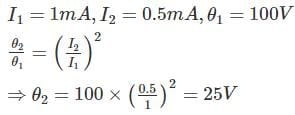QUESTION: 7

Deflection produced in Moving iron instruments is:

Solution:

The deflection in Moving iron instrument is given by,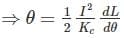Deflection is directly proportional to square of rms value of operating current

QUESTION: 8

A standard cell of 1.0185 V is used with a slide wire potentiometer. The balance was obtained at 60 cm. When an unknown emf was connected, the balance was obtained at 82 cm. What is the magnitude of unknownemf?

Solution:

Given that,

Standard voltage Es = 1.0185V

The balance obtained at 60 cm.

Resistance Rs = 60Ω

Working current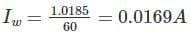Voltage corresponding to 82 cm,

V = 0.0169 × 82 = 1.39V =

QUESTION: 9

The type of wattmeter commonly used for measurement of power in AC circuits is:

Solution:

Dynamometer type instrument as wattmeter is used for the measurement of power in AC circuits

Moving iron type, rectifier type and thermocouple type instruments are mostly used as ammeter or voltmeter

QUESTION: 10

According to Blondel’s theorem, if a network is supplied using ‘n’ conductor’s the total power is ________ of the reading of n wattmeter’s so arranged that a current coil of each wattmeter is in each line and the corresponding pressure coil is connected between that line and a common point

Solution:

According to Blondel’s theorem the number of wattmeters to be required to measure the total power in n-phase system is either N or(N-1).

When separate neutral wire is available in the system the no. of watt meters to be required is N.

When the neutral wire is not available in the system, then the no. of watt meters to be required is (N-1). One line is acts as a common line for return path.

The total power is the sum of the all wattmeter readings.

QUESTION: 11

The disc of domestic supply energy meter is made of which material?

Solution:

The disc of domestic supply energy meter is made of aluminium.

Energy meter consists of rotating aluminium disc mounted on a spindle between two electro magnets.

QUESTION: 12

In a single phase induction type energy meter, the deflecting torque is________the true power in the circuit.

Solution:

In a single phase induction type energy meter, the deflecting torque is directly proportional to the true power in the circuit.

QUESTION: 13

The dead time of an instrument refers to _____.

Solution:

Dead Time: It is the time before the instrument begins to response after the quantity has altered

Dead Space (or) Dead Zone: In some instruments, for some range of input values, there is no change in output. This range of input is called dead space (or) dead zone.

QUESTION: 14

A piezometer is used to measure:

Solution:

A piezometer is a device used to measure liquid pressure in a system by measuring the height to which a column of the liquid rises against gravity. It is used to measure very low pressures.

QUESTION: 15

The potentiometer wires should have _______:

Solution:

The potentiometer wires should have high resistivity (specific resistance) and low temperature coefficient. The alloys constantan and manganin are traditionally used for this purpose.

QUESTION: 16

The vertical gain control of CRO is set at a deflection factor of 5 V/cm. An unknown sinusoidal is applied to Y-input. A 20 cm long straight line trace is observed, what will the ac voltage be?

Solution:

Deflection sensitivity is defined as,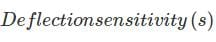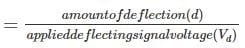Deflection factor is the reciprocal of deflection sensitivity.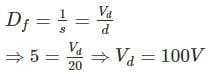QUESTION: 17

Which meter is suitable for the measurement of 10 mV at 50 MHz?

Solution:

CRO is used to measure the quantities at higher frequencies. Hence CRO is suitable for the measurement of 10 mV at 50 MHz.

QUESTION: 18

Which of the following is not suitable for the measurement of low resistances?

Solution:

Kelvin’s double bridge is used for the measurement of very low resistances. Using potentiometer method and Ammeter Voltmeter method we can measure low resistance.

Wheatstone bridge is suitable for the measurement of medium resistance

QUESTION: 19

Which is the most suitable thermocouple transducer for the measurement of temperature in the range of 1300°C to 1500°C?

Solution: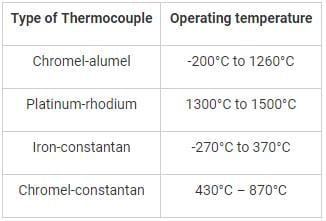QUESTION: 20

Capacitive transducers are normally used for:

Solution:

Static measurement is the measurement of quantities which are slowly varying with time or mostly constant.

Dynamic measurement is the measurement of quantities which changes rapidly with time.

Capacitive transducers are used for dynamic measurements, used for measuring the displacement, pressure and other physical quantities.Use Code STAYHOME200 and get INR 200 additional OFF Use Coupon Code